General Question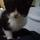Can some one tell me how to solve any of these precal equations?

Asked by monsoon (2510) March 30th, 2008

I am trying to pass an online test to get into a college calculus class, and I took precal like three years ago. These are the questions I got wrong and I need to figure out why. I have the answers, but I don’t understand how to reach them mathematically.

Also, I’ve written equations in a sort of long form because I don’t have proper symbols, so exponents are written as x^y, like on a calculator.

Thanks to anyone who’ll help me with this, I really need it.

1. Find the value of the side of a square whose diagonal is known as ‘a’. (Answer: [a times (square root of 2)]/2)

2. evaluate: sin of pi/6 (Answer: 1/2)

3. A population has P(t)=155063e^(0.14t) individuals, how man days (t) are necessary for the population to reach 1,000,000? (Answer: 13.3137)

4. distance of a car is represented by s=t^2+2t Find the average velocity from t=1 to t=5. (Answer: 12ft/sec)

Observing members: 0Composing members: 0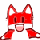1. Find the value of the side of a square whose diagonal is known as ‘a’. (Answer: [a times (square root of 2)]/2)
think about the square as 2 triangles, the diagonal is the hypotenuse. a^2+b^2=c^2, and since a=b, since its a square, 2a^2=c^2. take the square root of both sides, a*sqrt(2), which equals a+b. since they’re equal, you divide it in two, thuse the (a*sqrt(2))/2)

2. evaluate: sin of pi/6 (Answer: 1/2)
unit circle, the x is cos, y is sin

3. A population has P(t)=155063e^(0.14t) individuals, how man days (t) are necessary for the population to reach 1,000,000? (Answer: 13.3137)
its a problem of continuous interest. e= 2.71828182, set the equation equal to 1,000,000, and solve using the natural log.

4. distance of a car is represented by s=t^2+2t Find the average velocity from t=1 to t=5. (Answer: 12ft/sec)
this one i’ll have to get back to you on. what does everything stand for; does s = distance and t = velocity, or does t = time?

skwerl88 (532)“Great Answer” (1) Flag as…awesome, but I’m sorry,

4. s= distance, and t= time

monsoon (2510)“Great Answer” (0) Flag as…that makes things easier; try some physics formulas in there. d=vt for instance.

skwerl88 (532)“Great Answer” (0) Flag as…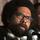purple

okc405 (255)“Great Answer” (1) Flag as…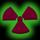4. This one you’ll need a bit of calculus. First take your function t^2+2t, take the first derivative of it: d/dx f(x)=x^n + c = nx^n-1 +c: d/dt = 2t+2. V(t)=s’(t). Evaluate the derivative at t=1 and t=5. Find the average. (V(1) + V(5)) / 2.

winblowzxp (498)“Great Answer” (0) Flag as…At t=5 V(t) = 12, so the average velocity can’t be 12. 12 is the max for that interval.

winblowzxp (498)“Great Answer” (0) Flag as…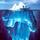You can solve #4 without calculus. (This is, after all, pre-calc !)

According to the given formula:
At t=5 seconds the car has gone a distance of s = 5^2 + 2*5 = 35 feet
At t=1 second, the car was at a distance of s = 1^2 + 2 = 3 feet.
Therefore, in the time interval from t = 1 to 5,
the car moved a total distance of 35 – 3 = 32 feet
taking a total time of 5 – 1 = 4 seconds.

Average speed = (total distance) / (total time)
= 32 ft / 4 sec = 8 ft/sec

gasman (11315)“Great Answer” (0) Flag as…or